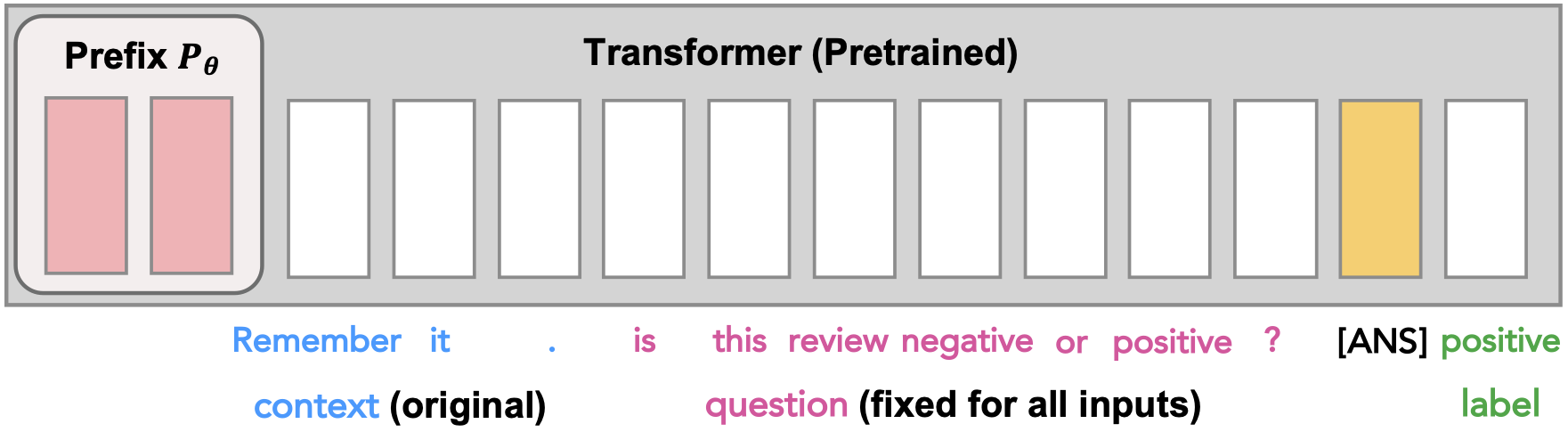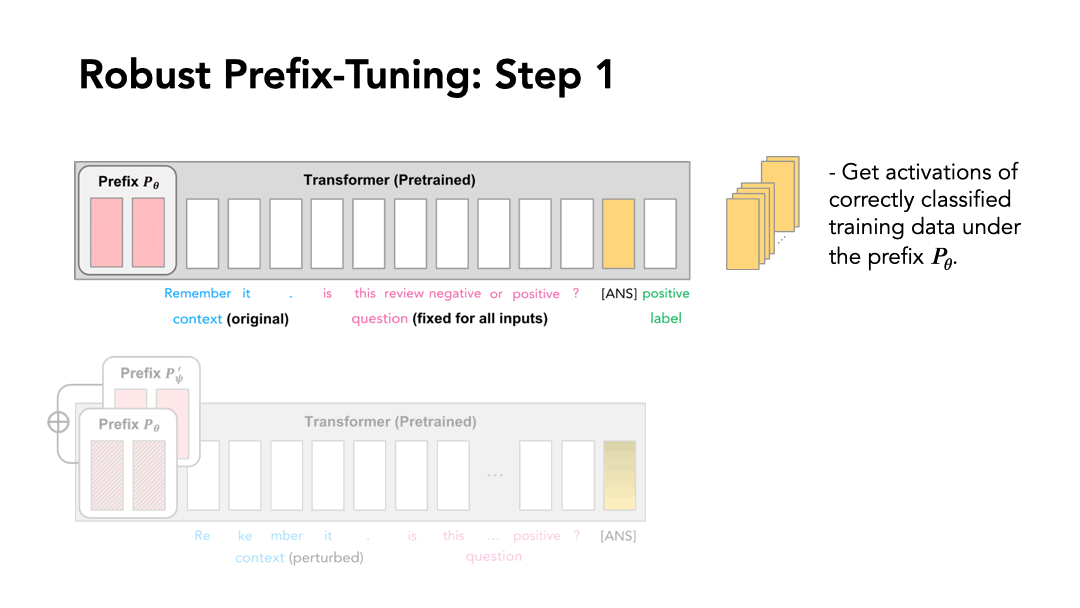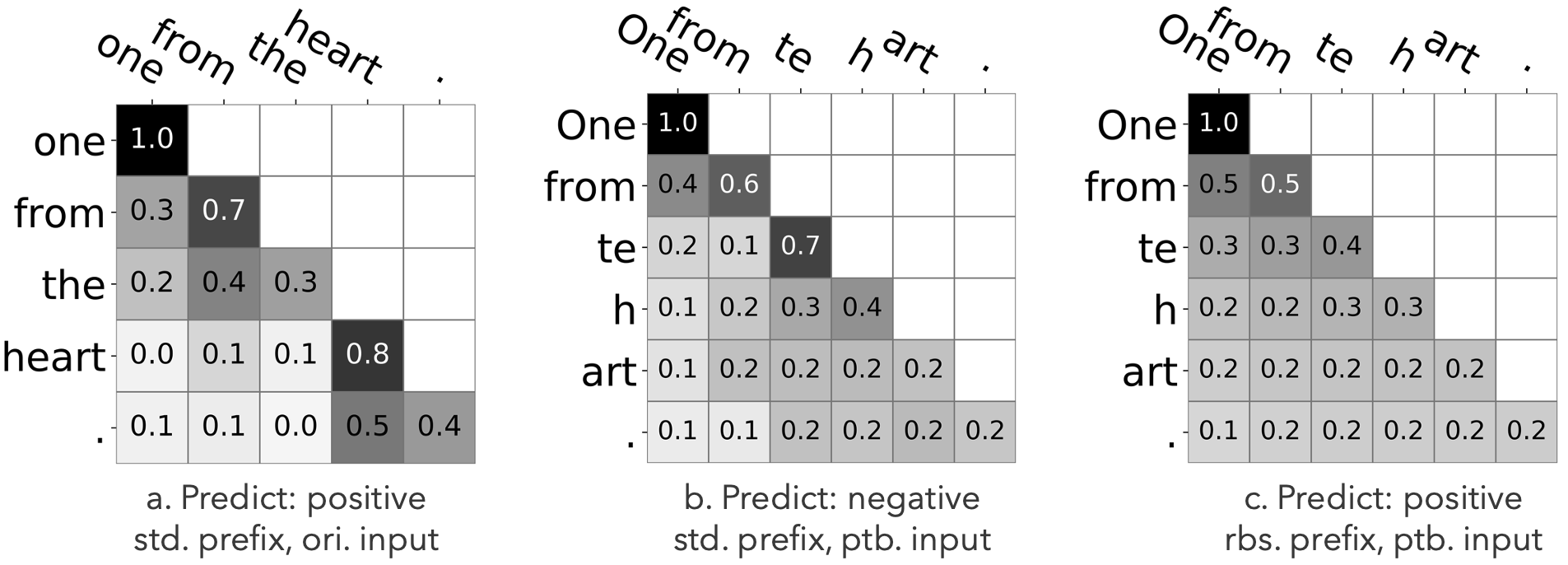Prefix-tuning (Li & Liang, 2021) is going viral. Instead of finetuning the entire large-scale language model, it is demonstrated that you just need to tune a prefix when adapting to a certain downstream task. There are indeed many merits in prefix-tuning: more expressive than discrete prompts (which is enabled by continuous optimization), lightweight compared with finetuning (near 1000x fewer parameters to be updated), as well as modular (one prefix one task). Without a doubt, prefix-tuning will motivate us to consider more about adapting tasks to the pretrained LMs in this era of parameter-efficient finetuning (Liu et al., 2021; Ding et al., 2022).

We experimented with prefix-tuning on text classification tasks. Despite all the merits in prefix-tuning, we found it lacking in robustness. It’s easy to fool the prefix by manipulating the inputs (see an example below). In hindsight, perhaps we shouldn’t be surprised by such phenomenon (Ilyas et al., 2019) - Defending against adversarial attacks for prefix-tuning is what we are gonna do now, as we want parameter-efficient finetuning techniques to be robust.But we got really surprised when looking at the current defense methods in NLP. Roughly speaking, four types of defense methods have been proposed: model functional improvement (Li & Sethy, 2019; Jone et al.,2020), robustness certification (Jia et al., 2019; Shi et al., 2020), adversary detection (Pruthi et al., 2019; Zhou et al., 2019), as well as adversarial training (Miyato et al., 2017; Miyato et al., 2019; Zhu et al., 2020; Yi et al., 2021). There are many more papers not listed here, but most of the techniques require modification to the architecture and the parameters of the LM or additional maintenance of adversary detectors.

Directly applying such techniques necessitates auxiliary model update and storage, which will inevitably hamper the modularity of prefix-tuning. It seems that adversarial training is the only exception, but it is notorious for its excessively long training time (and we’ll soon find it not suitable in the prefix-tuning scenario). We ask the following question:

Can we improve the robustness of prefix-tuning while preserving its efficiency and modularity, without modifying the pretrained model parameters?

Our answer is YES. Let me show you what we do in our ICLR 2022 paper.

## Designing Robust Prefixes

How do we achieve both robustness and parameter efficiency?

To answer this question, we first observe how prefix-tuning steers a GPT-style LM to classify a sentence.Causal LMs cast the text classification problem as label generation. When classifying, the LM generates the predicted label token at the output position (with a special token [ANS] as input) after autoregressively processing all tokens in the sentence. Now focus on the output position: while the input token is the same ([ANS]), the output prediction token differs according to different contexts.To be more specific, it’s the affected layerwise activation of the LM that results in different model bahaviour at the output position. As a result, we might want to rectify the erroneous activation in case the model is fooled.

So how do we rectify the activation? Recall that prefix-tuning steers the activation of LMs. In the same vein, we can expect a prefix to rectify the erroneous activation when optimized properly. In our work, we propose to tune another prefix $$P'_\Psi$$ to achieve this during inference, with the original prefix $$P_\theta$$ fixed. We expect the added prefix $$P_\theta + P'_\Psi$$ will steer correct activations under attacks.

Now all we need is the additional prefix for robustness: no need to modify the pretrained LM, which preserves the parameter efficiency. To tune the additional prefix $$P'_\Psi$$, we assume that all the correct activations at layer $$j$$ lie on a manifold $$\mathcal{M}^{(j)}$$, and propose to minimize the orthogonal component of an erroneous activation. Our method is illustrated as follows:We construct the canonical manifold by PCA to characterize the layerwise activation of the correctly-classified inputs. For the $$j$$-th layer, we obtain a layerwise linear projection matrix $$Q^{(j)}$$. When prompted with $$P_\theta + P'_\Psi$$ (with $$P'_\Psi$$ initialized as $$\mathbf{0}$$), the $$j$$-th layer activation at the output position $$o$$ is $$h_{o}^{(j)}$$, and

$\| h_{o}^{(j)} - h_{o}^{(j)} Q^{(j)} \|_2$

defines the loss function to update $$P'_\Psi$$ on the fly.

## Performance of the Robust Prefix

We implement both standard and adversarial training to obtain the original prefix $$P_\theta$$ and experiment on three datasets: SST-2, AG’s News, and SNLI. With properly tuned robust prefix $$P'_\Psi$$, the robustness of both standard and adversarial prefix-tuning is significantly improved. Check the results from SST-2 for example:See more results in our paper. It is also noted that standard prefix-tuning, when equipped with the robust prefix, outperforms adversarial prefix-tuning without robust prefix in terms of robustness (right bar in the left figure v.s. orange bar in the right figure). This suggests that our robust prefix-tuning might be a better solution compared with adversarial training. We are further convinced of this when comparing standard and adversarial prefix-tuning:As you can find in the left figure, adversarial prefix-tuning achieves slightly better epoch-wise convergence and generalization. With the x-axis replaced with clocktime, however, it is shown that adversarial prefix-tuning requires much longer time to converge.

## Behavior Analysis

The summed prefix $$P_\theta + P'_\Psi$$ steers the correct activation of the LM, which dramatically improves the robustness of prefix-tuning. Compared with the original prefix $$P_\theta$$, how does the robust prefix steer the LM?

By visualizing the attention map in the top layer, we find that the robust prefix averages the attention under in-sentence attacks.Take the sentence from SST-2 development set as an example. The original input is one from the heart . Under the TextBugger attack, the input is perturbed into One from te h art . and the attention map is perplexed. With the robust prefix, the attention is averaged for each token. As a result, the robust prefix have steered the top layer of the LM to behave like a continous Bag of Words (cBoW) model. Roughly speaking, we can infer that the averaged attention as leads to equal contribution of each token in the input to the final prediction. In this way, the LM will not concentrate on some malicious perturbed tokens (e.g., the te in the middle figure). This is, however, only a less rigorous interpretation as attention can be not that reliable to be an explanation. But anyway, the attention in the top layer of the LM becomes averaged with our robust prefix.Take another example from SST-2 dev set. The original input is it ‘s just filler . The adversarial trigger is lifts mates who. This time, we calculate a type of token importance based on the attention map for more reliable explanations (as suggested in previous work; check our paper for details). According to the middle figure, the LM attaches much importance to the trigger tokens, which results in mistaken prediction. With the robust prefix, it is shown that the importance is reallocated to the essential token filler. As a result, the robust prefix helps ignore the distraction under UAT attack.

We have also conducted quantitative analysis to show these two findings are statistically significant. Again, check our paper for details :)

## Takeaway Message

We tune an additional prefix (which preserves the strengths of the original prefix-tuning method) during inference (which avoids the computational cost of adversarial training) to steer correct activation (refining the representation at the output position in the pretrained LM).

Our code is available at https://github.com/minicheshire/Robust-Prefix-Tuning. Future work includes but is not limited to constructing a better canonical manifold, generalizing the method to text generation, etc.